Here is a beautiful picture of the contents of one of our litter boxes: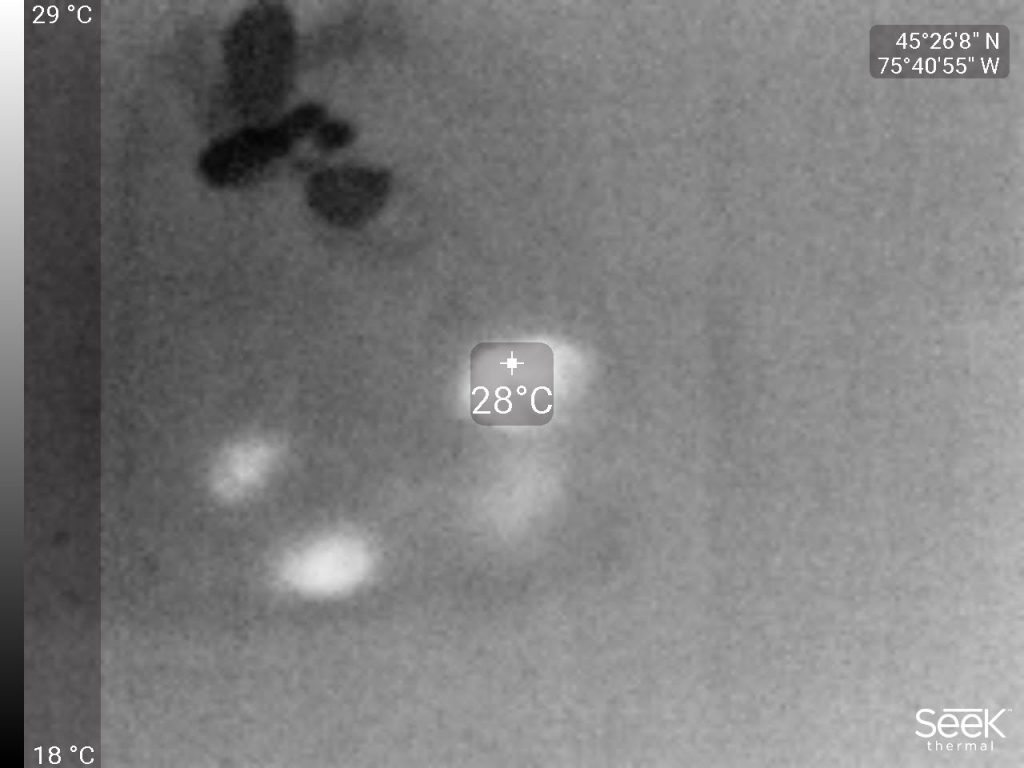What makes it beautiful, you ask? Why, it is a thermal infrared image. And it shows some unambiguously fresh poop. Fresh poop produced just a few minutes prior by our kitty Pipacs, whom we were about to write off two weeks ago, as we were certain that his digestive system shut down, him being unable to poop as his bowels are obstructed by an invasive bone growth.

But Pipacs still has a few of his nine little lives left. I don’t know how long he’ll stay with us; we take it one day at a time. But with the medication he receives (a combination of laxatives and stool softeners) he is coping for the time being.

I was surprised by the number of people who found my little exercise about kinetic energy interesting.

However, I was disappointed by the fact that only one person (an astrophysicist by trade) got it right.

It really isn’t a very difficult problem! You just have to remember that in addition to energy, momentum is also conserved.

In other words, when a train accelerates, it is pushing against something… the Earth, that is. So ever so slightly, the Earth accelerates backwards. The change in velocity may be tiny, but the change in energy is not necessarily so. It all depends on your reference frame.

So let’s do the math, starting with a train of mass $$m$$ that accelerates from $$v_1$$ to $$v_2$$. (Yes, I am doing the math formally; we can plug in the actual numbers in the end.)

Momentum is of course velocity times mass. Momentum conversation means that the Earth’s speed will change as

$\Delta v = -\frac{m}{M}(v_2-v_1),$

where $$M$$ is the Earth’s mass. If the initial speed of the earth is $$v_0$$, the change in its kinetic energy will be given by

$\frac{1}{2}M\left[(v_0+\Delta v)^2-v_0^2\right]=\frac{1}{2}M(2v_0\Delta v+\Delta v^2).$

If $$v_0=0$$, this becomes

$\frac{1}{2}M\Delta v^2=\frac{m^2}{M}(v_2-v_1)^2,$

which is very tiny if $$m\ll M$$. However, if $$|v_0|>0$$ and comparable in magnitude to $$v_2-v_1$$ (or at least, $$|v_0|\gg|\Delta v|$$), we get

$\frac{1}{2}M(2v_0\Delta v+\Delta v^2)=-mv_0(v_2-v_1)+\frac{m^2}{2M}(v_2-v_1)^2\simeq -mv_0(v_2-v_1).$

Note that the actual mass of the Earth doesn’t even matter; we just used the fact that it’s much larger than the mass of the train.

So let’s plug in the numbers from the exercise: $$m=10000~{\rm kg}$$, $$v_0=-10~{\rm m}/{\rm s}$$ (negative, because relative to the moving train, the Earth is moving backwards), $$v_2-v_1=10~{\rm m}/{\rm s}$$, thus $$-mv_0(v_2-v_1)=1000~{\rm kJ}$$.

So the missing energy is found as the change in the Earth’s kinetic energy in the reference frame of the second moving train.

Note that in the reference frame of someone standing on the Earth, the change in the Earth’s kinetic energy is imperceptibly tiny; all the $$1500~{\rm kJ}$$ go into accelerating the train. But in the reference frame of the observer moving on the second train on the parallel tracks, only $$500~{\rm kJ}$$ goes into the kinetic energy of the first train, whereas $$1000~{\rm kJ}$$ is added to the Earth’s kinetic energy. But in both cases, the total change in kinetic energy, $$1500~{\rm kJ}$$, is the same and consistent with the readings of the electricity power meter.

Then again… maybe the symbolic calculation is too abstract. We could have done it with numbers all along. When a $$10000~{\rm kg}$$ train’s speed goes from $$10~{\rm m}/{\rm s}$$ to $$20~{\rm m}/{\rm s}$$, it means that the $$6\times 10^{24}~{\rm kg}$$ Earth’s speed (in the opposite direction) will change by $$10000\times 10/(6\times 10^{24})=1.67\times 10^{-20}~{\rm m}/{\rm s}$$.

In the reference frame in which the Earth is at rest, the change in kinetic energy is $$\tfrac{1}{2}\times (6\times 10^{24})\times (1.67\times 10^{-20})^2=8.33\times 10^{-16}~{\rm J}$$.

However, in the reference frame in which the Earth is already moving at $$10~{\rm m}/{\rm s}$$, the change in kinetic energy is $$\tfrac{1}{2}\times (6\times 10^{24})\times (10+1.67\times 10^{-20})^2-\tfrac{1}{2}\times (6\times 10^{24})\times 10^2$$$${}=\tfrac{1}{2}\times (6\times 10^{24})\times[2\times 10\times 1.67\times 10^{-20}+(1.67\times 10^{-20})^2]$$$${}\simeq 1000~{\rm kJ}$$.

Enough blogging about personal stuff like our cats. Here is a neat little physics puzzle instead.

Solving this question requires nothing more than elementary high school physics (assuming you were taught physics in high school; if not, shame on the educational system where you grew up). No tricks, no gimmicks, no relativity theory, no quantum mechanics, just a straightforward application of what you were taught about Newtonian physics.

We have two parallel rail tracks. There is no friction, no air resistance, no dissipative forces.

On the first track, let’s call it A, there is a train. It weighs 10,000 kilograms. It is accelerated by an electric motor from 0 to 10 meters per second. Its kinetic energy, when it is moving at $$v=10~{\rm m/s}$$, is of course $$K=\tfrac{1}{2}mv^2=500~{\rm kJ}$$.

Next, we accelerate it from 10 to 20 meters per second. At $$v=20~{\rm m/s}$$, its kinetic energy is $$K=2000~{\rm kJ}$$, so an additional $$1500~{\rm kJ}$$ was required to achieve this change in speed.

All this is dutifully recorded by a power meter that measures the train’s electricity consumption. So far, so good.

But now let’s look at the B track, where there is a train moving at the constant speed of $$10~{\rm m/s}$$. When the A train is moving at the same speed, the two trains are motionless relative to each other; from B‘s perspective, the kinetic energy of A is zero. And when A accelerates to $$20~{\rm m/s}$$ relative to the ground, its speed relative to B will be $$10~{\rm m/s}$$; so from B‘s perspective, the change in kinetic energy is $$500~{\rm kJ}$$.

But the power meter is not lying. It shows that the A train used $$1500~{\rm kJ}$$ of electrical energy.

Question: Where did the missing $$1000~{\rm kJ}$$ go?

Concerned as we were (and still are) about the health of our smallest kitty Pipacs, it helps to remember that we have two other felines in the house, both in good health as far as we know (fingers crossed and all that.)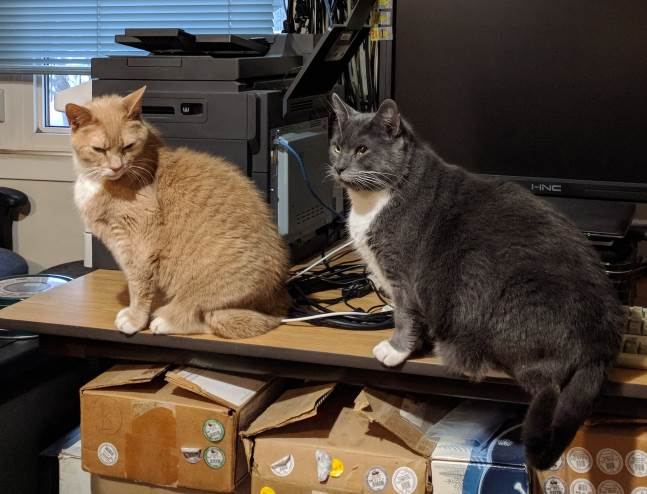The orange tabby on the left, Kifli, will turn 17 in April. He will be old enough to vote in next year’s federal election! He has not stated his political preference yet.

And despite their similarities, the two cats are not related. The cat on the right, Rufus, was a stray. He was approximately one year old when we adopted him in the fall of 2014.

Much to our surprise, not to mention relief, our kittycat Pipacs is a lot better today.

We noticed yesterday afternoon: he began eating again. He seemingly felt better. He groomed himself. He even played with us a little.

And then this morning: a nice, big poop in the litter box.

So his digestive system is functioning again. Probably with difficulty, so he is going to be permanently on a diet of laxatives and stool softeners, but so long as he is able to poop, he should be okay as otherwise, other than the bone growth on his pelvis, he is healthy.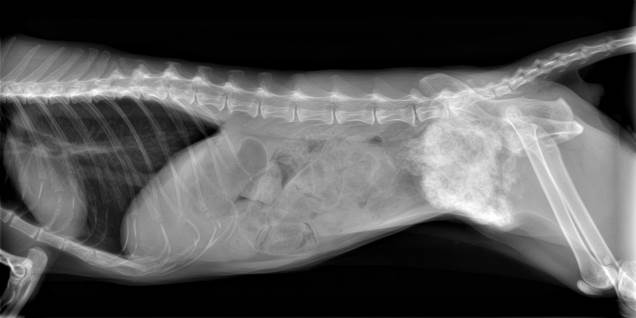This bone growth is not going to go away, so we’re living on borrowed time. But borrowed time is still a lot better than no time at all.

I already called the vet and canceled the scheduled visit for tonight. Thinking of Murphy’s law, however, I asked them not to process a refund yet, just keep the amount we already paid on our account.

This beautiful creature, who has been our companion for ten and a half years, give or take, is Pipacs (Hungarian for Poppy, pronounced pi-patch or something like, with the ‘i’ as in the word hit, the ‘a’ in the word bark, with emphasis on the first syllable.)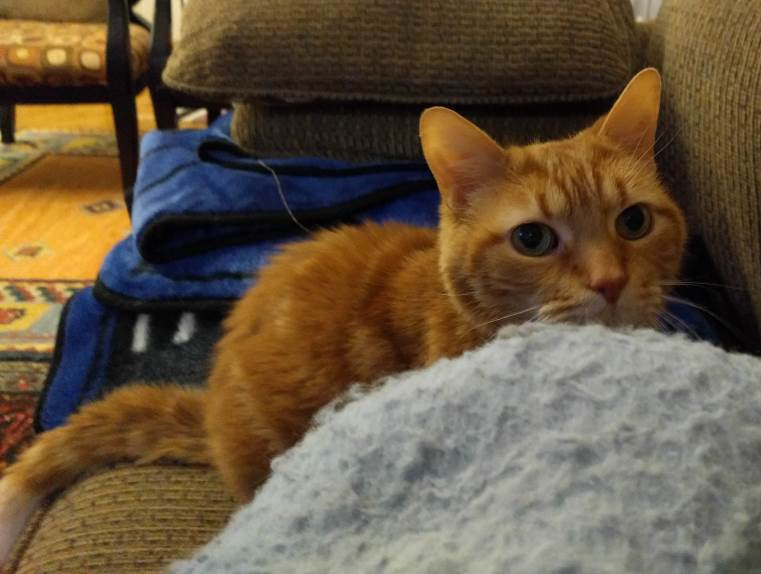Unfortunately, Pipacs is very ill.

He was diagnosed with a growth on his pelvis three years ago. Even back then, the only treatment option was drastic surgery: Removal of a large part of his pelvis along with the leg on that side.

Pipacs has always been a very skittish cat. He was a stray when we adopted him, probably about a year old in late summer 2007. He is very easily traumatized. This, and the very low risk of such a growth spreading to other organs led us to the decision not to opt for surgery.

One thing we did not anticipate is that the growth, which increased in size rather dramatically in the past few months, would encroach on his digestive system and eventually obstruct his colon.

Which is exactly what happened. Simply put, beyond incidents of explosive diarrhea after receiving enemas, Pipacs cannot poop anymore. And sadly, surgery is no longer a viable option.

Which is why our veterinarian is scheduled to make a home visit tomorrow with her euthanasia kit. And my heart breaks as I am writing these words.

Not too long ago (OK, well, 30 years… it doesn’t feel that long anymore) there was another genius on the news: the Genius of the Carpathians, Romanian dictator Nicolae Ceausescu.

Here is a joke from that era that, no doubt, could be adapted to fit the “very stable genius”, too.

The US president, the General Secretary of the Soviet communist party, the Pope and Ceausescu are flying somewhere on a plane. Suddenly, the pilot enters the compartment and says, “Gentleman, I have bad news. This airplane is about to crash and we only have four parachutes.”

Immediately, the US president yells, “I am the leader of the free world, I must survive!”, grabs a parachute and jumps.

He is followed by the Soviet leader, who yells, “I am the leader of the worldwide socialist revolution. I must survive!”, grabs another parachute and jumps.

Next comes Ceausescu: “I am the Genius of the Carpathians! I must survive!”, grabs the next parachute and jumps.

The Pope and the pilot remain. The Pope looks at the pilot and says, “My son, I am old and lived a full life. Your whole life is ahead of you. Please take that parachute. I’ll pray for your survival.” The pilot responds, “No need to worry, Holy Father, we have two parachutes left. The Genius of the Carpathians grabbed the fire extinguisher.”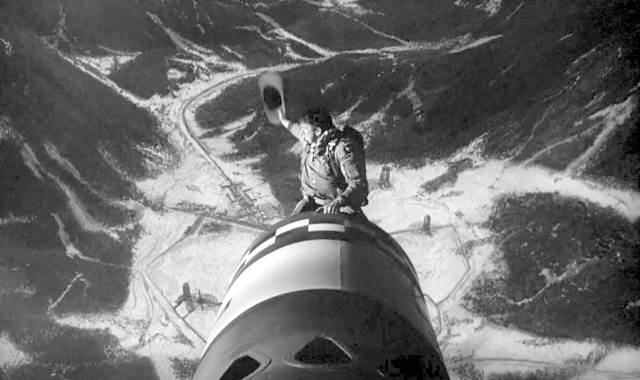When will news portals finally learn that autoplaying a video at maximum volume in the middle of the night guarantees only one thing: that I close the tab in a mad panic while I curse the news site, its creators, editors, their parents and grandparents and just about everybody they ever did business with for scaring me witless and waking up my household?